# Python – Blood Cell Identification using Image Processing

Detection of White Blood Cell and Red Blood Cell is very useful for various medical applications, like counting of WBC, disease diagnosis, etc. Circle detection is the most suitable approach. This article is the implementation of suitable image segmentation and feature extraction techniques for blood cell identification, on the obtained enhanced images.
Input :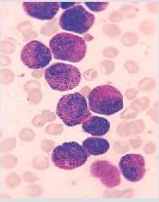Original Blood Smear Microscopic Image

Code: Python Code for Image Enhancement

 `import` `numpy as np ` `import` `cv2 ` `import` `matplotlib.pyplot as plt ` ` `  `# read original image ` `image ``=` `cv2.imread(``"c1.png"``) ` ` `  `# convet to gray scale image ` `gray ``=` `cv2.cvtColor(image, cv2.COLOR_BGR2GRAY) ` `cv2.imwrite(``'gray.png'``, gray) ` ` `  `# apply median filter for smoothning ` `blurM ``=` `cv2.medianBlur(gray, ``5``) ` `cv2.imwrite(``'blurM.png'``, blurM) ` ` `  `# apply gaussian filter for smoothning ` `blurG ``=` `cv2.GaussianBlur(gray, (``9``, ``9``), ``0``) ` `cv2.imwrite(``'blurG.png'``, blurG) ` ` `  `# histogram equalization ` `histoNorm ``=` `cv2.equalizeHist(gray) ` `cv2.imwrite(``'histoNorm.png'``, histoNorm) ` ` `  `# create a CLAHE object for  ` `# Contrast Limited Adaptive Histogram Equalization (CLAHE)  ` `clahe ``=` `cv2.createCLAHE(clipLimit ``=` `2.0``, tileGridSize``=``(``8``, ``8``)) ` `claheNorm ``=` `clahe.``apply``(gray) ` `cv2.imwrite(``'claheNorm.png'``, claheNorm) ` ` `  ` `  `# contrast stretching  ` `# Function to map each intensity level to output intensity level.  ` `def` `pixelVal(pix, r1, s1, r2, s2): ` `    ``if` `(``0` `<``=` `pix ``and` `pix <``=` `r1): ` `        ``return` `(s1 ``/` `r1) ``*` `pix ` `    ``elif` `(r1 < pix ``and` `pix <``=` `r2): ` `        ``return` `((s2 ``-` `s1) ``/` `(r2 ``-` `r1)) ``*` `(pix ``-` `r1) ``+` `s1 ` `    ``else``: ` `        ``return` `((``255` `-` `s2) ``/` `(``255` `-` `r2)) ``*` `(pix ``-` `r2) ``+` `s2 ` ` `  `    ``# Define parameters.  ` ` `  ` `  `r1 ``=` `70` `s1 ``=` `0` `r2 ``=` `200` `s2 ``=` `255` ` `  `# Vectorize the function to apply it to each value in the Numpy array.  ` `pixelVal_vec ``=` `np.vectorize(pixelVal) ` ` `  `# Apply contrast stretching.  ` `contrast_stretched ``=` `pixelVal_vec(gray, r1, s1, r2, s2) ` `contrast_stretched_blurM ``=` `pixelVal_vec(blurM, r1, s1, r2, s2) ` ` `  `cv2.imwrite(``'contrast_stretch.png'``, contrast_stretched) ` `cv2.imwrite(``'contrast_stretch_blurM.png'``,  ` `            ``contrast_stretched_blurM) ` ` `  `# edge detection using canny edge detector ` `edge ``=` `cv2.Canny(gray, ``100``, ``200``) ` `cv2.imwrite(``'edge.png'``, edge) ` ` `  `edgeG ``=` `cv2.Canny(blurG, ``100``, ``200``) ` `cv2.imwrite(``'edgeG.png'``, edgeG) ` ` `  `edgeM ``=` `cv2.Canny(blurM, ``100``, ``200``) ` `cv2.imwrite(``'edgeM.png'``, edgeM) `

Output Enhanced Images: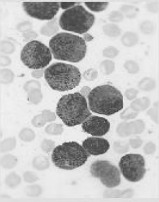Gray Scale Image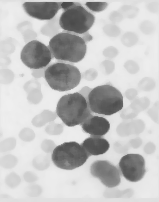Median Filtered Image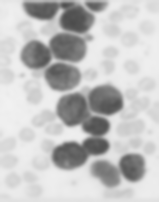Gaussian Filtered Image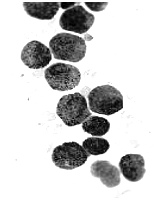Contrast Stretched Image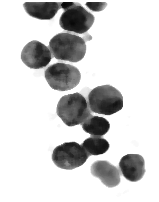Contrast Stretching on Median Filtered Image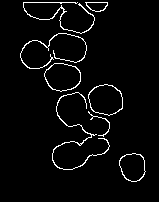Canny Edge Detection on Gaussian Filtered Image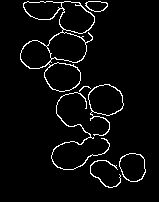Canny Edge Detection on Median Filtered Image

Image Segmentation and Feature Extraction

 `# read enhanced image  ` `img ``=` `cv2.imread(``'cell.png'``, ``0``) ` ` `  `# morphological operations ` `kernel ``=` `np.ones((``5``, ``5``), np.uint8) ` `dilation ``=` `cv2.dilate(img, kernel, iterations ``=` `1``) ` `closing ``=` `cv2.morphologyEx(img, cv2.MORPH_CLOSE, kernel) ` ` `  `# Adaptive thresholding on mean and gaussian filter ` `th2 ``=` `cv2.adaptiveThreshold(img, ``255``, cv2.ADAPTIVE_THRESH_MEAN_C, \ ` `            ``cv2.THRESH_BINARY, ``11``, ``2``) ` `th3 ``=` `cv2.adaptiveThreshold(img, ``255``, cv2.ADAPTIVE_THRESH_GAUSSIAN_C, \ ` `            ``cv2.THRESH_BINARY, ``11``, ``2``) ` `# Otsu's thresholding ` `ret4, th4 ``=` `cv2.threshold(img, ``0``, ``255``, cv2.THRESH_BINARY ``+` `cv2.THRESH_OTSU) ` ` `  `# Initialize the list ` `Cell_count, x_count, y_count ``=` `[], [], [] ` ` `  `# read original image, to display the circle and center detection   ` `display ``=` `cv2.imread(``"D:/Projects / ImageProcessing / DA1 / sample1 / cellOrig.png"``) ` ` `  `# hough transform with modified circular parameters ` `circles ``=` `cv2.HoughCircles(image, cv2.HOUGH_GRADIENT, ``1.2``, ``20``,  ` `                           ``param1 ``=` `50``, param2 ``=` `28``, minRadius ``=` `1``, maxRadius ``=` `20``) ` ` `  `# circle detection and labeling using hough transformation  ` `if` `circles ``is` `not` `None``: ` `        ``# convert the (x, y) coordinates and radius of the circles to integers ` `        ``circles ``=` `np.``round``(circles[``0``, :]).astype(``"int"``) ` ` `  `        ``# loop over the (x, y) coordinates and radius of the circles ` `        ``for` `(x, y, r) ``in` `circles: ` ` `  `                ``cv2.circle(display, (x, y), r, (``0``, ``255``, ``0``), ``2``) ` `                ``cv2.rectangle(display, (x ``-` `2``, y ``-` `2``),  ` `                              ``(x ``+` `2``, y ``+` `2``), (``0``, ``128``, ``255``), ``-``1``) ` `                ``Cell_count.append(r) ` `                ``x_count.append(x) ` `                ``y_count.append(y) ` `        ``# show the output image ` `        ``cv2.imshow(``"gray"``, display) ` `        ``cv2.waitKey(``0``) ` ` `  `# display the count of white blood cells  ` `print``(``len``(Cell_count)) ` `# Total number of radius ` `print``(Cell_count)  ` `# X co-ordinate of circle ` `print``(x_count)      ` `# Y co-ordinate of circle ` `print``(y_count)      `

Output Images: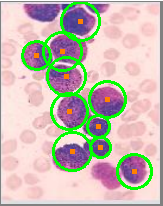Blood Cell Detectionq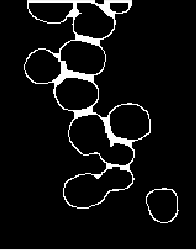Closing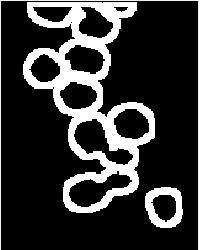Dilation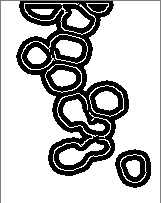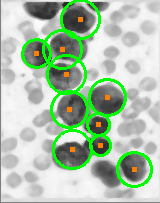Modified Haugh Transformation for circle detection

My Personal Notes arrow_drop_upCheck out this Author's contributed articles.

If you like GeeksforGeeks and would like to contribute, you can also write an article using contribute.geeksforgeeks.org or mail your article to contribute@geeksforgeeks.org. See your article appearing on the GeeksforGeeks main page and help other Geeks.

Please Improve this article if you find anything incorrect by clicking on the "Improve Article" button below.

Article Tags :

1

Please write to us at contribute@geeksforgeeks.org to report any issue with the above content.